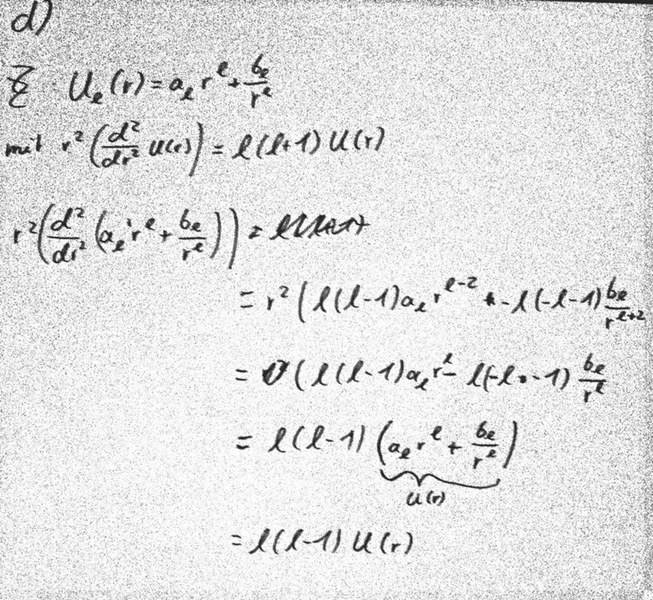# Solution of the radial part of the laplace-equation

## Homework Statement

I got the the radial part of the Laplace-Equation:

$r^2(\frac{d^2}{dr^2}U(r))=l(l+1)U(r)$

Now I should show that the following solves the equation:

$a_l*r^l+\frac{b_l}{r^l}$

## The Attempt at a SolutionThe problem is that I got l(l-1) instead of l(l+1) :(

TSny
Homework Helper
Gold Member
Hello, and welcome to PF!

## Homework Statement

I got the the radial part of the Laplace-Equation:

$r^2(\frac{d^2}{dr^2}U(r))=l(l+1)U(r)$

The expression on the left is incorrect. See here for Laplace's equation in spherical coordinates.

Sorry for the late answer. the ansatz was wrong, instead of $a_r*r^l+\frac{b_l}{r^l}$ it has to be:

$a_r*r^l+\frac{b_l}{r^{l+1}}$